# - 9th grade (14y) - math problems

#### Number of problems found: 3135

• Day trip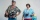A group of tourists went on a day trip. For two hours they walked at a speed of 6 km/h, for another hour they climbed a steep hill at a speed of 2 km/h, spent an hour and a half at lunch, then in an hour they inspected the castle garden at a free step at
• TaxesIf you were charged \$243 in taxes on a \$4,050 purchase, what percent tax were you charged?
• Sale goods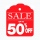Sale goods were reduced twice. First by 15% and then by another 20% of the new price. What percentage of the original price was the final price?
• A diesel carA diesel car costs \$2599 more than an unleaded petrol car and travels an average of 25,000km per year. Diesel petrol has a consumption rate of 7.4L per 100km and costs \$1.39 per litre. Unleaded petrol has a consumption rate of 8.5L per 100km and costs \$1.
• The sum 16The sum of A and B is 36. The difference of A & B is 8. find the larger number of A.
• Insurance 2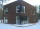A house that is valued at 255,000 euros has been insured for 224,000 euro. At what percent has it been insured?
• The sum 15The sum of the real numbers x and y is 24. Their difference is 12. What is the value of xy?
• Comparing exponents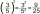A) 8 (6²)(8²) b) 16 (6²)(8²) c) (6¹+²) (8²)
• Regular price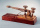The sale price of a television set is 7200. The discount rate is 40%. Find its regular price.
• The average 4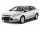The average cost price of a car in Ireland is now €26,430 excluding VAT. If VAT is 23% calculate the actual selling price of a car in 2021.
• The sum 13The sum of the exponents of the prime factors in the prime factorization of 196 is. .. .
• Sum and productThe sum of the real numbers x and y is 12. Their difference is 8. What is the value of xy?
• Points OPQ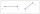Point P is on line segment OQ. Given OP = 6, OQ = 4x - 3, and PQ = 3x, find the numerical length of OQ.
• TrapezoidsIn the isosceles trapezoid ABCD we know: AB||CD, |CD| = c = 8 cm, height h = 7 cm, |∠CAB| = 35°. Find the area of the trapezoid.
• Find a 2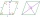Find a length of the diagonal AC of the rhombus ABCD if its perimeter P = 112 dm and the second diagonal BD has a length of 36 dm.
• One of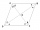One of the internal angles of the rhombus is 120° and the shorter diagonal is 3.4 meters long. Find the perimeter of the rhombus.
• The ratio 4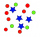The ratio of two number is 5:4 if 40% of the first number is 12, what will be 50% of the second number?
• Length 9Length of a rectangle is 8 m less than twice of breadth. If the perimeter of the rectangle is 50. find its length and breadth.
• Cost structure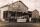You are currently trying to decide between two cost structures for your business: one that has a greater proportion of short-term fixed costs and another that is more heavily weighted to variable costs. Estimated revenue and cost data for each alternative
• Dimensions of rectangleFind the dimensions of a rectangle given that its perimeter is 110 cm and its length is 1 cm more than twice its width.

Do you have an exciting math question or word problem that you can't solve? Ask a question or post a math problem, and we can try to solve it.

We will send a solution to your e-mail address. Solved examples are also published here. Please enter the e-mail correctly and check whether you don't have a full mailbox.

Please do not submit problems from current active competitions such as Mathematical Olympiad, correspondence seminars etc...